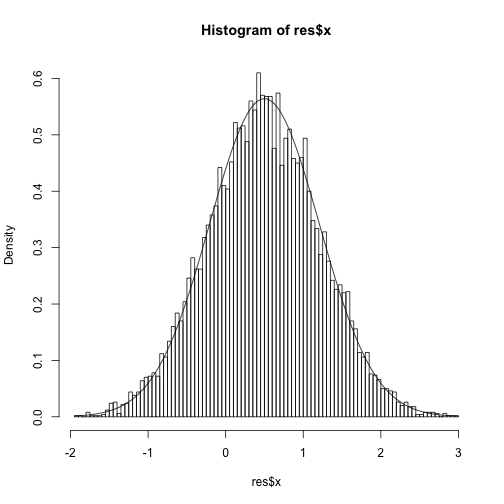This post is a demonstration of slice sampling. The goal is to sample from a target distribution f(x). A slice variable, u, is added where the joint density is p(u,x) = I(0<u<f(x)) which maintains the marginal distribution for x. A Gibbs sampler is constructed with the following steps

1.  u x ~ Unif(0,f(x))
2.  x u ~ Unif over the set A where A={x:u

The rest of this post considers situations where A is relatively easy to find, e.g. monotone functions f(x) over the support of x and unimodal functions.

Here is a generic function (okay, it probably isn’t that generic, but it works for the purpose below) to implement slice sampling. It takes a number of draws n, an initial x, the target density, and a function A that returns the interval A, so only unimodal targets supported. The function returns the samples x and u (for demonstration).

In this first example, the target distribution is Exp(1). The set A is just the interval (0,-log(u)) where u is the current value of the slice variable.

 Here is a demonstration of a single step of the slice sampler. Given a coordinate (u,x), the sampler first draws from u x which is equivalent to slicing the density vertically at x and drawing uniformly on the y-axis from the interval where u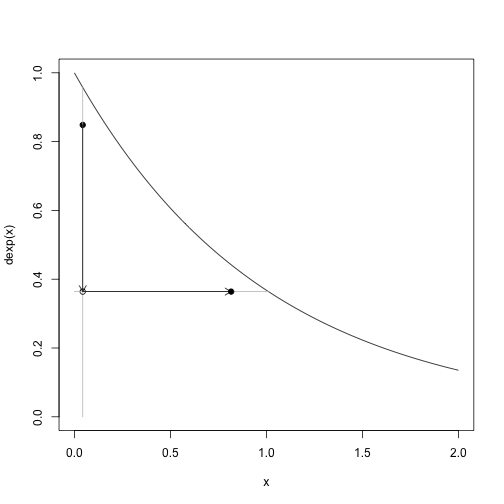Here are 9 steps, 10 samples including the intial, of the slice sampler: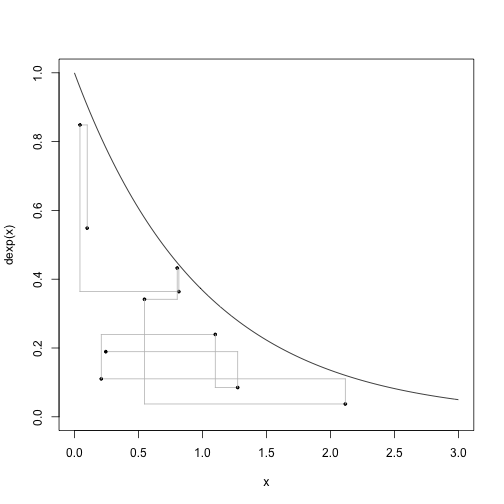And this is comparing the marginal draws for x to the truth.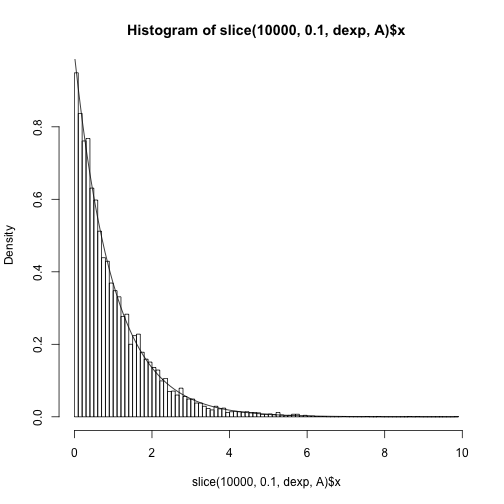Now I turn to a standard normal distribution where we pretend we cannot invert the density and therefore need another approach. The approach is going to be to use numerical methods to find the interval A (so, again assuming a unimodal target). The magic happens below where the `uniroot` function is used to find the endpoints of the interval.

Run the sampler for a standard normal target.

This is what one step looks like.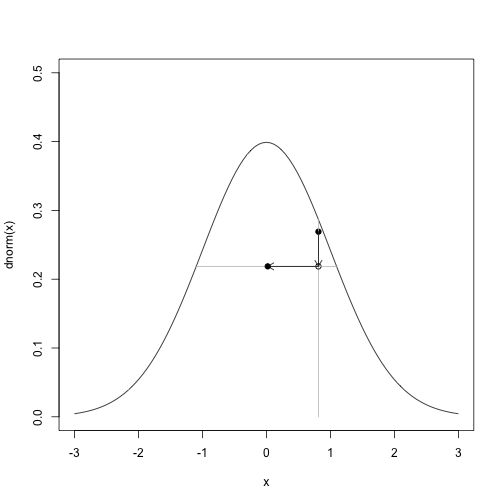Or 9 steps: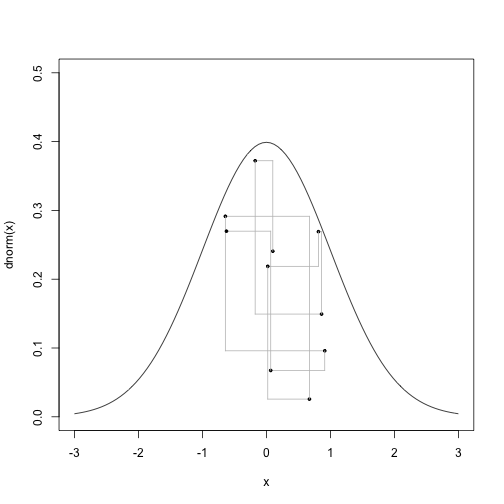And the fit to the target distribution.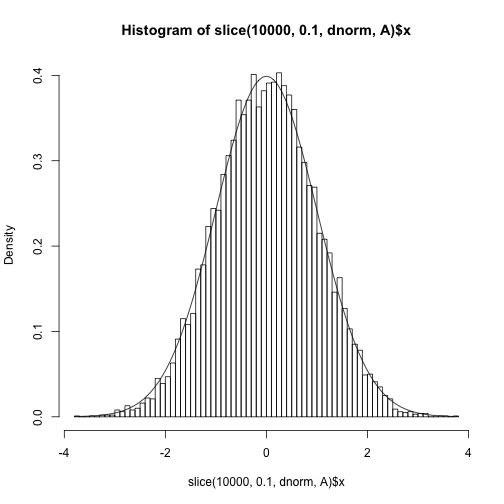## Using an unnormalized density

Slice sampling can also be performed on the unnormalized density.## Learning the truncation points

 Here is an alternative version of the slice sampler that samples from a distribution other than uniform. The main purpose of introducing this is in Bayesian inference where the target distribution is the posterior, p(x y) \propto p(y x) p(x), and the augmentation is p(u,x)\propto p(x) I(0
 The truncation points are determined by u
 This is run on the model y x ~ N(x,1) and x~N(0,1) and y=1 is observed. The true posterior is N(y/2,1/2).